### 机器学习07：朴素贝叶斯应用(词集模式)

• 发布时间：2017年3月8日 18:09
• 作者：杨仕航

#### 1、构建词汇表

```#coding:utf-8
import numpy as np

def create_dataset():
posting_list = [
'my dog has flea problems help please',
'maybe not take him to dog park stupid',
'my dalmation is so cute I love him',
'stop posting stupid worthless garbage',
'mr licks ate my steak how to stop him',
'quit huying worthless dog food stupid'
]
posting_list = map(lambda x:x.split(' '), posting_list)

#上面句子对应的性质，0代表非侮辱性句子，1代表侮辱性句子
class_vect = [0, 1, 0, 1, 0, 1]
return posting_list, class_vect```

```#根据训练集生成词汇表
def create_vocab_list(posting_list):
'''对所有句子取并集，获取词汇表'''
return list(reduce(lambda x,y:x|set(y), [set([])] + posting_list))```

#### 2、句子数字化（向量化）

```def set_of_words_to_vect(vocab_list, input_set):
'''对比句子向量和词汇表，找到对应出现在词汇表的位置'''
return map(lambda x: 1 if x in input_set else 0, vocab_list)```

```if __name__ == '__main__':
#创建训练集
posting_list, class_vect = create_dataset()

#获取词汇表
vocab_list = create_vocab_list(posting_list)

#输出词汇表
print(u'词汇表：')
print(vocab_list)
print('\n')

#测试句子数字化结果
posting = 'I love cute dog'.split(' ')
print(posting)
posting_vect = set_of_words_to_vect(vocab_list, posting)
print(posting_vect)```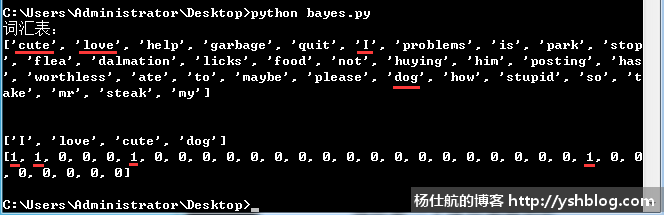#### 3、从词向量计算条件概率p(w|ci) p(ci)

= p(w1, w2, ..., wn|ci) p(ci)

= p(w1|ci) p(w2|ci) ... p(wn|ci) p(ci)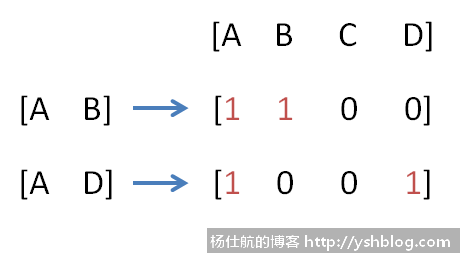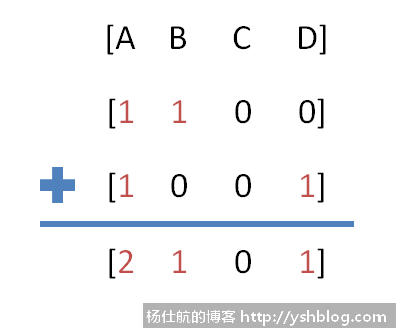ABCD4个单词对应条件概率为 [2/4, 1/4, 0/4, 1/4] = [0.5, 0.25, 0, 0.25]。

p(w|c0) p(c0)

= p('A'|c0) p('B'|c0) p('D'|c0) p(c0)

= 0.5*0.25*0.25*(2/5)

= 0.0125

p(w|c1) p(c1)

= p('A'|c1) p('B'|c1) p('D'|c1) p(c1)

= 1/6*1/6*1/6*(3/5)

≈ 0.0028

ps：这里我故意没有选择单词C组成句子，因为单词C在非侮辱性的情况概率为0。计算概率过程中乘以0会导致结果也为0。而且细心的同学会发现通常计算概率的结果都是小数点后好几位。这些情况下面代码会调整处理。

#### 4、计算条件概率代码

《机器学习实战》书中的代码比较乱，且不通用。我重新整理如下：

```#训练函数
def train_nb0(posting_vects, train_classes):
num_train_docs = len(posting_vects) #总句子数
num_words = len(posting_vects)   #词汇表单词数

#遍历分类标签，统计对应类别的数量
p_class_num = {} #各类别句子数
p_vect_num = {}  #各类别各单词数

for i, class_type in enumerate(train_classes):
#累计每个类别的单词数（初始化每个类别单词数为1个，避免为0的情况）
p_vect_num[class_type] = p_vect_num.get(class_type, np.ones(num_words)) + posting_vects[i]

#累计每个类别的句子数
p_class_num[class_type] = p_class_num.get(class_type, 0) + 1

#计算每个类别的条件概率，对应单词数除以该类别的单词总数
p_class = {} #各类别的概率
p_vect = {}  #各类别的各单词条件概率

for class_type in train_classes:
p_vect[class_type] = p_vect_num[class_type]/np.sum(p_vect_num[class_type])
p_class[class_type] = p_class_num[class_type]/float(num_train_docs)
return p_vect, p_class```

1）参数postring_vects是训练集的6个句子数字化组合的列表；参数train_classes是每个句子对应分类的列表。

2）第12行，统计每个类别的单词数时，初始化为1

```if __name__ == '__main__':
#创建训练集
posting_list, class_vect = create_dataset()

#获取词汇表
vocab_list = create_vocab_list(posting_list)

#句子单词数字化
posting_vects = []
for posting in posting_list:
posting_vect = set_of_words_to_vect(vocab_list, posting)
posting_vects.append(posting_vect)

#获取对应类别各单词的条件概率和各类别概率
p_vect, p_class = train_nb0(np.array(posting_vects), np.array(class_vect))

print(p_vect)
print(p_class)```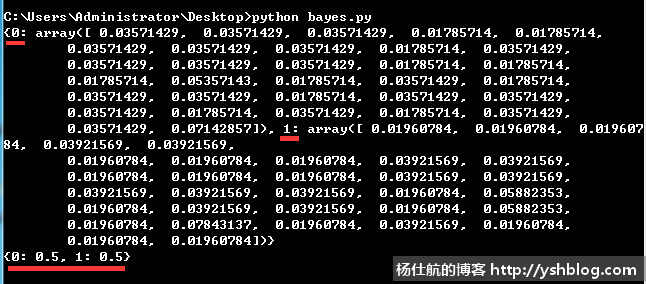#### 5、朴素贝叶斯分类函数

```#朴素贝叶斯分类函数
def classify_nb(vect_classify, p_vect, p_class):
#计算句子属于各类别的概率
p = {}
for class_type, vect in p_vect.items():
p[class_type] = np.sum(vect_classify * np.log(vect)) + np.log(p_class[class_type])

#获取最大概率的类别
return max(p.items(), key=lambda x:x)```

vect_classify是一个句子数字化之后的词向量。只有两种值0和1，直接乘以条件概率可以保留对应单词的条件概率。去掉不相干单词的条件概率。

```def testing_nb(test):
#创建训练集
posting_list, class_vect = create_dataset()

#获取词汇表
vocab_list = create_vocab_list(posting_list)

#句子单词数字化
posting_vects = []
for posting in posting_list:
posting_vect = set_of_words_to_vect(vocab_list, posting)
posting_vects.append(posting_vect)

p_vect, p_class = train_nb0(np.array(posting_vects), np.array(class_vect))

#测试句子
test_list = test.split(' ')
vect_test = set_of_words_to_vect(vocab_list, test_list)
return classify_nb(vect_test, p_vect, p_class)```

```if __name__ == '__main__':
print(testing_nb('love my dalmation'))
print(testing_nb('stupid garbage'))```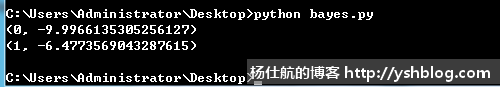《机器学习实战》相关的代码：

https://github.com/pbharrin/machinelearninginaction/blob/master/Ch04/bayes.py

```#coding:utf-8
import numpy as np

#创建训练集
def create_dataset():
posting_list = [
'my dog has flea problems help please',
'maybe not take him to dog park stupid',
'my dalmation is so cute I love him',
'stop posting stupid worthless garbage',
'mr licks ate my steak how to stop him',
'quit huying worthless dog food stupid'
]
posting_list = map(lambda x:x.split(' '), posting_list)

#上面句子对应的性质，0代表非侮辱性句子，1代表侮辱性句子
class_vect = [0, 1, 0, 1, 0, 1]
return posting_list, class_vect

#根据训练集生成词汇表
def create_vocab_list(posting_list):
'''对所有句子取并集，获取词汇表'''
return list(reduce(lambda x,y:x|set(y), [set([])] + posting_list))

#句子向量化
def set_of_words_to_vect(vocab_list, input_set):
'''对比句子向量和词汇表，找到对应出现在词汇表的位置'''
return map(lambda x: 1 if x in input_set else 0, vocab_list)

#训练函数
def train_nb0(posting_vects, train_classes):
num_train_docs = len(posting_vects) #总句子数
num_words = len(posting_vects)   #词汇表单词数

#遍历分类标签，统计对应类别的数量
p_class_num = {} #各类别句子数
p_vect_num = {}  #各类别各单词数

for i, class_type in enumerate(train_classes):
#累计每个类别的单词数（初始化每个类别单词数为1个，避免为0的情况）
p_vect_num[class_type] = p_vect_num.get(class_type, np.ones(num_words)) + posting_vects[i]

#累计每个类别的句子数
p_class_num[class_type] = p_class_num.get(class_type, 0) + 1

#计算每个类别的条件概率，对应单词数除以该类别的单词总数
p_class = {} #各类别的概率
p_vect = {}  #各类别的各单词条件概率

for class_type in train_classes:
p_vect[class_type] = p_vect_num[class_type]/np.sum(p_vect_num[class_type])
p_class[class_type] = p_class_num[class_type]/float(num_train_docs)
return p_vect, p_class

#朴素贝叶斯分类函数
def classify_nb(vect_classify, p_vect, p_class):
#计算句子属于各类别的概率
p = {}
for class_type, vect in p_vect.items():
p[class_type] = np.sum(vect_classify * np.log(vect)) + np.log(p_class[class_type])

#获取最大概率的类别
return max(p.items(), key=lambda x:x)

def testing_nb(test):
#创建训练集
posting_list, class_vect = create_dataset()

#获取词汇表
vocab_list = create_vocab_list(posting_list)

#句子单词数字化
posting_vects = []
for posting in posting_list:
posting_vect = set_of_words_to_vect(vocab_list, posting)
posting_vects.append(posting_vect)

p_vect, p_class = train_nb0(np.array(posting_vects), np.array(class_vect))

#测试句子
test_list = test.split(' ')
vect_test = set_of_words_to_vect(vocab_list, test_list)
return classify_nb(vect_test, p_vect, p_class)

if __name__ == '__main__':
print(testing_nb('love my dalmation'))
print(testing_nb('stupid garbage'))```